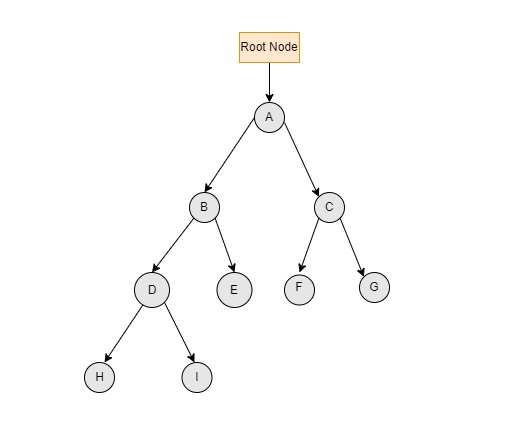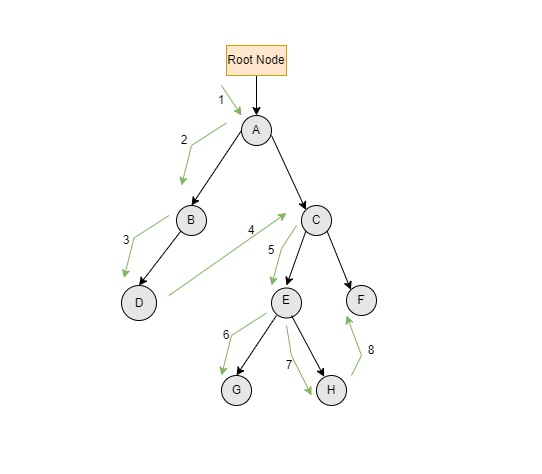# Pre-order traversal in a Javascript Tree

Tree is a hierarchical data structure which includes nodes and edges to it. Edges in tree acts as links connecting two nodes.

The Preorder tree traversal is a technique where the root node will be traversed first and then it will traverse the left subtree followed by the right subtree.

It will represent as

ROOT → LEFT → RIGHT


### Algorithm

These are the following steps to perform preorder tree traversal −

• Traverse the root node.

• Then, traverse the left subtree.

• Then, traverse the right subtree.

To understand the preorder traversal better, consider the following binary search tree −The traversal will start from the root node A and prints it. From there it visits its left subtree B and prints it. And again will print left subtree D. And again print the H subtree. Since, H does not have any left subtree further, H is printed. Then it will visit I which is right subtree of D. It goes on traversing in the same way until each and every node is visited.

The output of the above preorder tree traversal is –
A → B → D → H → I → E → C → F → G


Let's use the tree below as an example, adding new nodes to it.The above figure shows the path of preorder tree traversal represented using green arrows.

The above mentioned binary tree will produce the following output −
A→ B → D → C → E → G → H → F


## Example 1 (Using Recursion)

The following code provides a description of the Pre-order tree traversal with recursion technique.

<!DOCTYPE html>
<html>
<title>Pre-Order traversal of a tree</title>
<p id = "para"> </p>
<script>
class Node {
constructor(value) {
this.key = value;
this.left = null;
this.right = null;
}
}
function TreePreorder(node) {
if (node == null)
return;
document.write(node.key + " → ");
TreePreorder(node.left);
TreePreorder(node.right);
}
root = new Node('A');
root.left = new Node('B');
root.right = new Node('C');
root.left.left = new Node('D');
root.left.right = new Node('E');
root.left.left.left = new Node('H');
root.left.left.right = new Node('I');
root.right.left = new Node('F');
root.right.right = new Node('G');
document.getElementById("para").innerHTML = "Pre-order traversal of binary tree will be: " + TreePreorder(root);
</script>
</html>


## Output

The output of the above script will be −

Pre-order traversal of binary tree will be:
A → B → D → H → I → E → C → F → G →

## Example 2 (Using Stack)

Another way to traverse tree using pre-order technique can be done with the help of a stack.

<!DOCTYPE html>
<html>
<p id = "para"> </p>
<script>
class Node {
constructor(Node_value) {
this.data = Node_value;
this.right = null;
this.left = null;
}
}
let root;
function Stack_Preorder(node) {
if (node == null) {
return;
}
let node_Stack = [];
node_Stack.push(root);
while (node_Stack.length > 0) {
let mynode = node_Stack[node_Stack.length - 1];
document.write(mynode.data + " ");
node_Stack.pop();
if (mynode.right != null) {
node_Stack.push(mynode.right);
}
if (mynode.left != null) {
node_Stack.push(mynode.left);
}
}
}
// Putting values into the nodes of the tree
root = new Node('A');
root.left = new Node('B');
root.right = new Node('C');
root.left.left = new Node('D');
root.left.right = new Node('E');
root.right.left = new Node('H');
root.right.right = new Node('I');
root.left.left.right = new Node('G');
root.left.left.left = new Node('F');
Stack_Preorder(root);
</script>

A B D F G E C H I